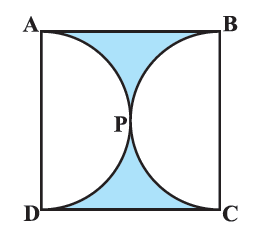# Ex.12.3 Q3 Areas Related to Circles Solution - NCERT Maths Class 10

## Question

Find the area of the shaded region in the given figure, if $$ABCD$$ is a square of side $$\text{14 cm}$$ and $$APD$$ and $$BPC$$ are semicircles.Video Solution
Areas Related To Circles
Ex 12.3 | Question 3

## Text Solution

What is known?

$$ABCD$$ is a square of side ($$l$$) $$\text{14 cm.}$$ $$APD$$ and $$BPC$$ are semicircles.

What is unknown?

Area of the shaded region.

Reasoning:

(i) From figure it is clear that diameter of both semicircles $$=$$ side of square $$=\text{14 cm}$$

$$\therefore \;$$ Radius of each semicircle \begin{align} (r) = \frac{{14}}{2} = 7{\text{cm}}\end{align}

(ii) Visually, it is clear

Area of shaded region = Area of square $$ABCD\, -$$ (Area of semicircle $$APD\, +$$ Area of semicircle $$BPC$$)

\begin{align} &= {\left( \rm{side} \right)^2} - \left( {\frac{{\pi {r^2}}}{2} + \frac{{\pi {r^2}}}{2}} \right)\\ &= {\left( \rm{side} \right)^2} - \pi {r^2}\end{align}

Steps:

Since semicircles $$APD$$ and $$BPC$$ are drawn using sides $$AD$$ and $$BC$$ respectively as diameter.

$$\therefore \;$$Diameter of each semicircle $$=\,\text{14 cm}$$

Radius of each semicircle \begin{align}(r) = \frac{{14}}{2} = 7{\text{cm}}\end{align}

Area of shaded region $$=$$ Area of square $$ABCD \,-$$ (Area of semicircle $$APD \,+$$Area of semicircle $$BPC$$)

\begin{align}&{ = {{({\text{side}})}^2} - \left( {\frac{1}{2}\pi {r^2} + \frac{1}{2}\pi {r^2}} \right)}\\&{ = {{14}^2} - \pi \times {{(7)}^2}}\\ &{ = 196 - \frac{{22}}{7} \times 7 \times 7}\\&{ = 196 - 154}\\&{ = 42\,{\text{c}}{{\text{m}}^2}}\end{align}

Learn from the best math teachers and top your exams

• Live one on one classroom and doubt clearing
• Practice worksheets in and after class for conceptual clarity
• Personalized curriculum to keep up with school